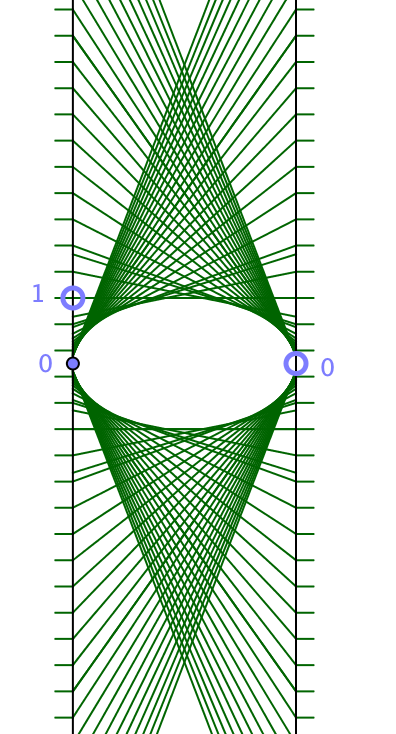# Function Diagram for y=1/x

Henri Picciotto

The input-output lines for the function y=1/x are all tangent to an ellipse, as you can see in this animation. (Click the 'play' ▷ button in the lower left hand corner of either pane. Clicking 'pause' ‖ will stop the animation.)

Here is a static diagram:In the particular case where the distance between the axes equals double the unit, the ellipse is a unit circle, and the result can be deduced from theorems in Euclidean geometry. See if you can work it out, using the figure:

AD=x, BE=1/x, QH is perpendicular to DE.
Prove DE is tangent to the circle by showing QH must be a radius.

Outline of a proof: Find the lengths of the sides of ∆QED. Conclude it is a right triangle. Calculate its area two ways to show that QH=1. Conclude that since the input-output line is perpendicular to a radius of the circle, through a point on the circle, it must be tangent to it. The algebra is somewhat complicated, but things simplify spectacularly.

There is also an alternate proof with easier calculations, using similar triangles.

The result can then be generalized to any distance between the axes through a horizontal stretch, which preserves tangency. Apparently, in projective geometry conics can be defined as sets of points, but also as the envelopes of families of lines. In this case, the ellipse in the left pane is the dual of the hyperbola in the right pane!